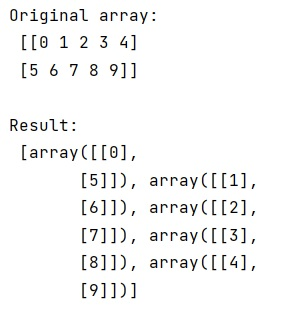# Slice 2d array into smaller 2d arrays

Learn, how to slice 2d array into smaller 2d arrays in Python?
Submitted by Pranit Sharma, on January 24, 2023

NumPy is an abbreviated form of Numerical Python. It is used for different types of scientific operations in python. Numpy is a vast library in python which is used for almost every kind of scientific or mathematical operation. It is itself an array which is a collection of various methods and functions for processing the arrays.

## Slicing 2d array into smaller 2d arrays

Suppose that we are given a two-dimensional array with more columns and we need to split this NumPy array into smaller two-dimensional arrays with fewer columns.

For this purpose, we will use the numpy.split() method where we will pass the total number of required sub-arrays that we want and the original array that has to be divided.

Let us understand with the help of an example,

## Python code to slice 2d array into smaller 2d arrays

```# Import numpy
import numpy as np

# Creating a numpy array
arr = np.arange(10).reshape([2,5])

# Display original array
print("Original array:\n",arr,"\n")

# Splitting array into 5 sub arrays
res = np.split(arr,5,axis=1)

# Display result
print("Result:\n",res,"\n")
```

Output:What's New (MCQs)

Top Interview Coding Problems/Challenges!

IncludeHelp's Blogs

Languages: » C » C++ » C++ STL » Java » Data Structure » C#.Net » Android » Kotlin » SQL
Web Technologies: » PHP » Python » JavaScript » CSS » Ajax » Node.js » Web programming/HTML
Solved programs: » C » C++ » DS » Java » C#
Aptitude que. & ans.: » C » C++ » Java » DBMS
Interview que. & ans.: » C » Embedded C » Java » SEO » HR
CS Subjects: » CS Basics » O.S. » Networks » DBMS » Embedded Systems » Cloud Computing
» Machine learning » CS Organizations » Linux » DOS
More: » Articles » Puzzles » News/Updates